﻿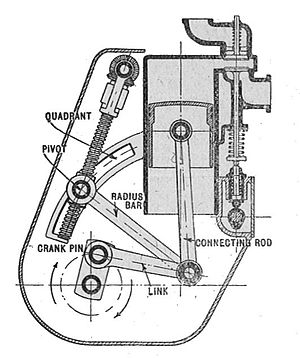Variable stroke engine (Autocar Handbook, Ninth edition)

A mechanical linkage is an assembly of bodies connected together to manage forces and movement. The movement of a body, or link, is studied using geometry so the link is considered to be rigid. The connections between links are modeled as providing ideal movement, pure rotation or sliding for example, and are called joints. A linkage modeled as a network of rigid links and ideal joints is called a kinematic chain.

Linkages may be constructed from open chains, closed chains, or a combination of open and closed chains. Each link in a chain is connected by a joint to one or more other links. Thus, a kinematic chain can be modeled as a graph in which the links are vertices and the joints are paths, which is called a linkage graph.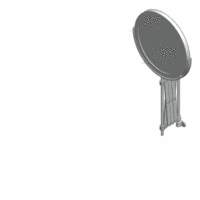The deployable mirror linkage is constructed from a series of rhombus or scissor linkages.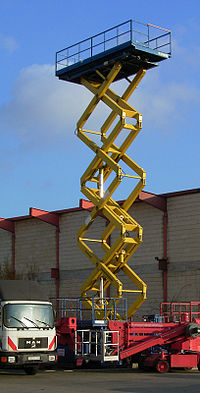Large scissor lift extended.

The movement of an ideal joint is generally associated with a subgroup of the group of Euclidean displacements. The number of parameters in the subgroup is called the degrees of freedom (DOF) of the joint.

Mechanical linkages are usually designed to transform a given input force and movement into a desired output force and movement. The ratio of the output force to the input force is known as the mechanical advantage of the linkage, while the ratio of the input speed to the output speed is known as the speed ratio. The speed ratio and mechanical advantage are defined so they yield the same number in an ideal linkage.

A kinimatic chain in which one link is fixed or stationary is called a mechanism, and a linkage designed to be stationary is called a structure.

## Uses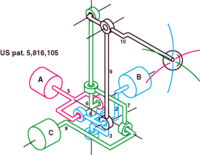A spatial 3 DOF linkage for joystick applications.

Perhaps the simplest linkage is the lever, which is a link that pivots around a fulcrum attached to ground, or a fixed point. As a force rotates the lever, points far from the fulcrum have a greater velocity than points near the fulcrum. Because power in to the lever equals the power out, a small force applied at a point far from the fulcrum (with greater velocity) equals a larger force applied at a point near the fulcrum (with less velocity). The amount the force is amplified is called mechanical advantage. This is the law of the lever.

Two levers connected by a rod so that a force applied to one is transmitted to the second is known as a four-bar linkage. The levers are called cranks, and the fulcrums are called pivots. The connecting rod is also called the coupler. The fourth bar in this assembly is the ground, or frame, on which the cranks are mounted.

Linkages are important components of machines and tools. Examples range from the four-bar linkage used to amplify force in a bolt cutter or to provide independent suspension in an automobile, to complex linkage systems in robotic arms and walking machines. The internal combustion engine uses a slider-crank four-bar linkage formed from it piston, connecting rod, and crankshaft to transform power from expanding burning gases into rotary power. Relatively simple linkages are often used to perform complicated tasks.

Interesting examples of linkages include the windshield wiper, the bicycle suspension, and hydraulic actuators for heavy equipment. In these examples the components in the linkage move in parallel planes and are called "planar linkages." A linkage with at least one link that moves in three dimensional space is called a "spatial linkage." The skeletons of robotic systems are examples of spatial linkages. The geometric design of these systems relies on modern computer aided design software.

The 4-bar linkage is an adapted mechanical linkage used on bicycles. With a normal full-suspension bike the back wheel moves in a very tight arc shape. This means that more power is lost when going uphill. With a bike fitted with a 4-bar linkage, the wheel moves in such a large arc that it is moving almost vertically. This way the power loss is reduced by up to 30%.

## History

Archimedes applied geometry to the study of the lever. Into the 1500s the work of Archimedes and Hero of Alexandria were the primary sources of machine theory. It was Leonardo da Vinci who brought an inventive energy to machines and mechanism.

In the mid-1700s the steam engine was of growing importance, and James Watt realized that efficiency could be increased by using different cylinders for expansion and condensation of the steam. This drove his search for a linkage that could transform rotation of a crank into a linear slide, and resulted in his discovery of what is called Watt's linkage. This led to the study of linkages that could generate straight lines, even if only approximately; and inspired the mathematician J. J. Sylvester, who lectured on the Peaucellier linkage, which generates an exact straight line from a rotating crank.

The work of Sylvester inspired A. B. Kempe, who showed that linkages for addition and multiplication could be assembled into a system that traced a given algebraic curve. Kempe's design procedure has inspired research at the intersection of geometry and computer science.

In the late 1800s F. Reuleaux and A. B. W. Kennedy and L. Burmester formalized the analysis and synthesis of linkage systems using descriptive geometry, and P.L.Chebyshev introduced analytical techniques for the study and invention of linkages.

In the mid 1900's F. Freudenstein and G. N. Sandor used the newly developed digital computer to solve the loop equations of a linkage and determine its dimensions for a desired function, initiating the computer-aided design of linkages. Within two decades these computer techniques were integral to the analysis of complex machine systems and the control of robot manipulators.

R.E.Kaufman combined the computer’s ability to rapidly compute the roots of polynomial equations with a graphical user interface to unite Freudenstein’s techniques with the geometrical methods of Reuleaux and Burmester and form KINSYN, an interactive computer graphics system for linkage design

The modern study of linkages includes the analysis and design of articulated systems that appear in robots, machine tools, and cable driven and tensegrity systems. These techniques are also being applied to biological systems and even the study of proteins.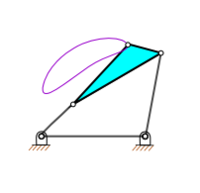Simple linkages are capable of producing complicated motion.

## Mobility

The configuration of a system of rigid links connected by ideal joints is defined by a set of configuration parameters, such as the angles around a revolute joint and the slides along prismatic joints measured between adjacent links. The geometric constraints of the linkage allow calculation of all of the configuration parameters in terms of a minimum set, which are the input parameters. The number of input parameters is called the mobility, or degree of freedom, of the linkage system.

A system of n rigid bodies moving in space has 6n degrees of freedom measured relative to a fixed frame. Include this frame in the count of bodies, so that mobility is independent of the choice of the fixed frame, then we have M=6(N-1), where N=n+1 is the number of moving bodies plus the fixed body.

Joints that connect bodies in this system remove degrees of freedom and reduce mobility. Specifically, hinges and sliders each impose five constraints and therefore remove five degrees of freedom. It is convenient to define the number of constraints c that a joint imposes in terms of the joint's freedom f, where c=6-f. In the case of a hinge or slider, which are one degree of freedom joints, we have f=1 and therefore c=6-1=5.

Thus, the mobility of a linkage system formed from n moving links and j joints each with fi, i=1, ..., j, degrees of freedom can be computed as,$M = 6n - \sum_{i=1}^j (6 - f_i) = 6(N-1 - j) + \sum_{i=1}^j\ f_i,$

where N includes the fixed link. This is known as Kutzbach-Gruebler's equation

There are two important special cases: (i) a simple open chain, and (ii) a simple closed chain. A single open chain consists of n moving links connected end to end by j joints, with one end connected to a ground link. Thus, in this case N=j+1 and the mobility of the chain is$M = \sum_{i=1}^j\ f_i .$

For a simple closed chain, n moving links are connected end-toend by n+1 joints such that the two ends are connected to the ground link forming a loop. In this case, we have N=j and the mobility of the chain is$M = \sum_{i=1}^j\ f_i - 6.$

An example of a simple open chain is a serial robot manipulator. These robotic systems are constructed from a series of links connected by six one degree-of-freedom revolute or prismatic joints, so the system has six degrees of freedom.

An example of a simple closed chain is the RSSR spatial four-bar linkage. The sum of the freedom of these joints is eight, so the mobility of the linkage is two, where one of the degrees of freedom is the rotation of the coupler around the line joining the two S joints.

### Planar and spherical movement

It is common practice to design the linkage system so that the movement of all of the bodies are constrained to lie on parallel planes, to form what is known as a planar linkage. It is also possible to construct the linkage system so that all of the bodies move on concentric spheres, forming a spherical linkage. In both cases, the degrees of freedom of the link is now three rather than six, and the constraints imposed by joints are now c=3-f.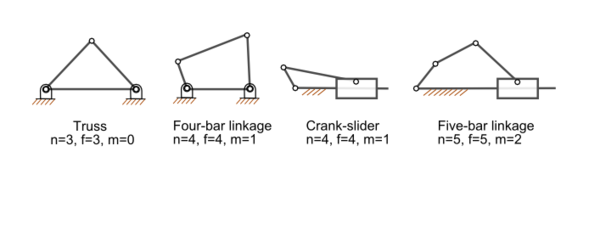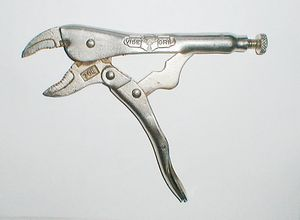Locking pliers exemplify a four-bar, one degree of freedom mechanical linkage. The adjustable base pivot makes this a two degree-of-freedom five-bar linkage.

In this case, the mobility formula is given by$M = 3(N- 1 - j)+ \sum_{i=1}^j\ f_i,$

and we have the special cases,

• planar or spherical simple open chain,$M = \sum_{i=1}^j\ f_i,$
• planar or spherical simple closed chain,$M = \sum_{i=1}^j\ f_i - 3.$

An example of a planar simple closed chain is the planar four-bar linkage, which is a four-bar loop with four one degree-of-freedom joints and therefore has mobility M=1.

## Joints

The most familiar joints for linkage systems are the revolute, or hinged, joint denoted by an R, and the prismatic, or sliding, joint denoted by a P. Most all other joints used for spatial linkages are modeled as combinations of revolute and prismatic joints. For example,

• the cylindric joint consists of an RP or PR serial chain constructed so that the axes of the revolute and prismatic joints are parallel,
• the spherical joint consists of an RRR serial chain for which each of the hinged joint axes intersect in the same point;
• the planar joint can be constructed either as a planar RRR, RPR, and PPR serial chain that has three degrees-of-freedom.

## Analysis and synthesis of linkages

The primary mathematical tool for the analysis of a linkage is known as the kinematics equations of the system. This is a sequence of rigid body transformation along a serial chain within the linkage that locates a floating link relative to the ground frame. Each serial chain within the linkage that connects this floating link to ground provides a set of equations that must be satisfied by the configuration parameters of the system. The result is a set of non-linear equations that define the configuration parameters of the system for a set of values for the input parameters.

Freudenstein introduced a method to use these equations for the design of a planar four-bar linkage to achieve a specified relation between the input parameters and the configuration of the linkage. Another approach to planar four-bar linkage design was introduced by L. Burmester, and is called Burmester theory.

The mobility formula provides a way to determine the number of links and joints in a planar linkage that yields a one degree-of-freedom linkage. If we require the mobility of a planar linkage to be M=1 and fi=1, the result is$M = 3(N-1-j) + j = 1, \!$

or$j = (3/2)N - 2. \!$

This formula shows that the linkage must have an even number of links, so we have

• N=2, j=1: this is a two-bar linkage known as the lever;
• N=4, j=4: this is the four-bar linkage;
• N=6, j=7: this is a six-bar linkage. A six-bar linkage two links that have three joints, called ternary links, and there are two topologies of this linkage depending how these links are connected. In the Watt topology, the two ternary are connected by a joint. In the Stephenson topology the two ternary links are connected by binary links;
• N=8, j=10: the eight-bar linkage has 16 different topologies;
• N=10, j=13: the 10-bar linkage has 230 different topologies,
• N=12, j=16: the 12-bar has 6856 topologies.

See Sunkari and Schmidt for the number of 14- and 16-bar topologies, as well as the number of linkages that have two, three and four degrees-of-freedom.

The planar four-bar linkage is probably the simplest and most common linkage. It is a one degree-of-freedom system that transforms an input crank rotation or slider displacement into and output rotation or slide.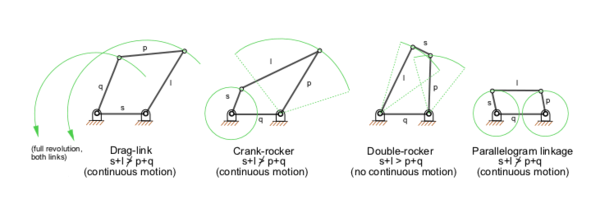• the crank-rocker, in which the input crank fully rotates and the output link rocks back and forth;
• the slider-drank, in which the input crank rotates and the output slide moves back and forth;
• drag-link mechanisms, in which the input crank fully rotates and drags the output crank in a fully rotational movement.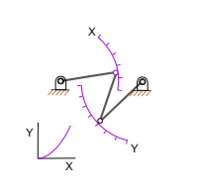A function generator linkage that approximates a parabolic output.

• Pantograph (four-bar, two DOF)
• Five bar linkages often have meshing gears for two of the links, creating a one DOF linkage. They can provide greater power transmission with more design flexibility than four-bar linkages.
• Klann linkage is a six-bar linkage that forms the leg of a walking mechanism;
• Toggle mechanisms are four-bar linkages that are dimensioned so that they can fold and lock. The linkage is dimensioned so that the linkage reaches a toggle position just before it folds. The high mechanical advantage allows the input crank to deform the linkage just enough push the it beyond the toggle position. This locks the input in place. Toggle mechanisms are used as clamps.

### Straight line mechanisms

• James Watt's parallel motion and Watt's linkage
• Peaucellier–Lipkin linkage, the first planar linkage to create a straight line output from rotary input; eight-bar, one DOF.
• A Scott Russell linkage, which converts linear motion, to (almost) linear motion in a line perpendicular to the input.
• Chebyshev linkage, which provides nearly straight motion of a point with a four-bar linkage.
• Hoekens linkage, which provides nearly straight motion of a point with a four-bar linkage.
• Sarrus linkage, which provides motion of one surface in a direction normal to another.

Linkage systems are widely distributed in animals. The most thorough overview of the different types of linkages in animals has been provided by M. Muller, who also designed a new classification system, which is especially well suited for biological systems. A well-known example is the cruciate ligaments of the knee.

An important difference between biological and engineering linkages is that revolving bars are rare in biology and that usually only a small range of the theoretically possible is possible due to additional mechanical constraints (especially the necessity to deliver blood). Biological linkages frequently are compliant. Often one or more bars are formed by ligaments, and often the linkages are three-dimensional. Coupled linkage systems are known, as well as five-, six-, and even seven-bar linkages. Four-bar linkages are by far the most common though.

Linkages can be found in joints, such as the knee of tetrapods, the hock of sheep, and the cranial mechanism of birds and reptiles. The latter is responsible for the upward motion of the upper bill in many birds.

Linkage mechanisms are especially frequent and manifold in the head of bony fishes, such as wrasses, which have evolved many specialized feeding mechanisms. Especially advanced are the linkage mechanisms of jaw protrusion. For suction feeding a system of linked four-bar linkages is responsible for the coordinated opening of the mouth and 3-D expansion of the buccal cavity. Other linkages are responsible for protrusion of the premaxilla.

Linkages are also present as locking mechanisms, such as in the knee of the horse, which enables the animal to sleep standing, without active muscle contraction. In pivot feeding, a four-bar linkage at first locks the head in a ventrally bent position by the alignment of two bars. The release of the locking mechanism jets the head up and moves the mouth toward the prey within 5-10 ms.

Wikimedia Foundation. 2010.

### Look at other dictionaries:

• Mechanical system — This article is about systems that manage mechanical movement. For other uses, see Machine (disambiguation). A mechanical system manages power to accomplish a task that involves forces and movement. Mechanical is derived from the Latin word… …   Wikipedia

• linkage — 1874, from LINK (Cf. link) (v.) + AGE (Cf. age). To understand the principle of Peaucellier s link work, it is convenient to consider previously certain properties of a linkage, (to coin a new and useful word of general application), consisting… …   Etymology dictionary

• linkage — /ling kij/, n. 1. the act of linking; state or manner of being linked. 2. a system of links. 3. Genetics. an association between two or more genes on a chromosome that tends to cause the characteristics determined by these genes to be inherited… …   Universalium

• mechanical brakes — Service brakes that are actuated by a mechanical linkage (rather than hydraulic lines) connecting the brakes to the brake pedal. The parking brake on most vehicles is mechanical …   Dictionary of automotive terms

• linkage — noun a) A mechanical device that connects things. A linkage in my cars transmission is broken so I cant shift out of first gear. b) A connection or relation between things or ideas. Syn: association, bond, connection, link, r …   Wiktionary

• mechanical system — noun a system of elements that interact on mechanical principles • Hypernyms: ↑system • Hyponyms: ↑fuel injection, ↑fuel injection system, ↑linkage, ↑lubricating system, ↑force feed lubricating system, ↑ …   Useful english dictionary

• Cognate linkage — In Kinematics, cognate linkages are linkages that ensure the same input output relationship or coupler curve geometry, while being dimensionally dissimilar. In case of four bar linkage coupler cognates, the Roberts–Chebychev theorem states that… …   Wikipedia

• Four bar linkage — A four bar linkage or simply a 4 bar or four bar is the simplest movable linkage. It consists of 4 rigid bodies (called bars or links), each attached to two others by single joints or pivots to form a closed loop. Four bars are simple mechanisms… …   Wikipedia

• Watt's linkage — (also known as the parallel linkage) is a type of mechanical linkage invented by James Watt (19 January 1736 ndash; 25 August 1819) to constrain the movement of a steam engine piston in a straight line. The idea of its genesis using links is… …   Wikipedia